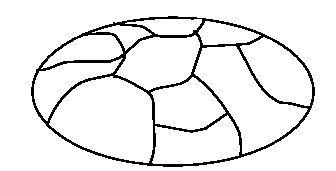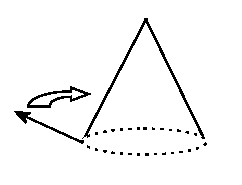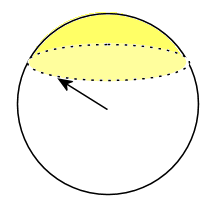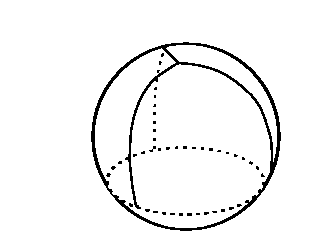Gauss-Bonnet Surface Description

The Gauss-Bonnet theorem is used to describe the total curvature of a closed surface.  A closed surface is one that does not have any boundaries, such as a sphere or a cube.  Although the mathematics behind Gauss-Bonnet theorem is quite complex the essence can be seen using the simple formulation:

KA = 2 π χ

Where:

K = Constant curvature

A = Area of the surface

χ = Euler number = 2 - 2*Genus of Surface (roughly the number of holes)

and

KA = Total curvature

To understand this we need to look at how curvature is measured and how the Euler number is calculated.

Any closed surface is may be divided up by drawing a number of faces on to it.  The Euler number is a relationship between the faces, edges and vertices:

χ = Faces – Edges + Vertices

Surprisingly this number does not change if you alter the number of faces.  For example in the curved shape below (figure 1) will always have an Euler number of 2, no matter how you divide it.Figure 1.

A domed surface with a flat underside.  If the flat under underside is one face then:

Faces = 12.  Edges = 29.  Vertices = 19.  Euler Number = 12 – 29 + 19 = 2.

In fact all of solids without holes in (cube, sphere, potato) will have a Euler number of 2.  For shapes with one hole in, such as a torus (a doughnut shape) the Euler number will always be 0.

Now for curvature. Let’s say we want to measure the curvature of the cone below (figure 2).  This is not a closed surface, it is an open ended cone where the surface ends at the boundary marked by the dotted line.  Now imagine an arrow projecting from the boundary of the cone and perpendicular to the cone’s surface.  Move this arrow around the boundary in a full circle.Figure 2.

Now imagine another identical arrow inside a sphere (figure 3), with the start of the arrow at the centre of the sphere and the tip of the arrow on the inner surface.   If this second arrow copies the rotation (but not the translation) of the first arrow it will trace a line on the surface of the sphere as in the figure below.Figure 3.

It must be noted that this is a unit sphere with a radius of 1 unit.  The area of the shaded yellow surface outlined by the arrow gives the Gaussian curvature of the cone.  If the yellow surface covers � of the unit sphere then the curvature (K) is �.  If it covers the whole surface (as it would if we were measuring the curvature of a sphere) the curvature is 1.  However, if we were to increase the radius of the sphere in figure 3, the same surface would cover a smaller area.  Thus the relationship between the curvature and the radius is given by:

K = 1 / r2

Given this it is possible to verify the Gauss-Bonnet equation above.  Let’s say we take a unit sphere and divide the surface into 4 triangles as in figure 4.Figure 4.

A unit sphere divided into 4 triangular faces.  This could also be considered a distorted tetrahedron.

Since this is a unit sphere then the curvature K is 1/r2. Substituting these values into the Gauss-Bonnet formula from above we get an Euler number of 2.  This marries up with the Eluer number calculated using the Euler formula defined earlier (4 – 6 + 4 = 2).

Since the total curvature is given by KA and we have both K and A (The surface area of a sphere is 4π r2) we can say:

 Total curvature = 1/ r2 * 4π r2 = 4π

Here the radius values cancel out so we do not need the radius to calculate the curvature of the sphere.  Therefore the curvature will always be a constant 4π, whatever the sphere’s size.

So we have a simple formula for calculating the total curvature of a sphere.  Now consider the fact that the Euler number is always going to be the same for a closed surface with no holes in it.  This means that all closed surfaces with no holes in will have a total curvature of 4π. Using this we can generalize to say we know the curvature of any closed shape.  For example take a torus.  This has an Euler number of 0.  Putting this Euler number into the Gauss-Bonnet equation we see that total curvature of a torus is 0.  Of course a torus is curved; this just means the net curvature is 0.  Since all shapes with one hole have an Euler number of 0 we can say that the total curvature for all these shapes is 0.  In fact we can extend the use of the equation to calculate the total curvature of any surface that we know the Euler number for.

It is clear that using the Gauss-Bonnet formula is far simpler than calculating curvature by imagining arrows that a draw surfaces on the inside of a sphere.  Moreover, using the Gauss-Bonnet formula it is possible to calculate the total curvature of any genus of shape (as long as it is a closed surface) just by counting the number of holes in that shape.